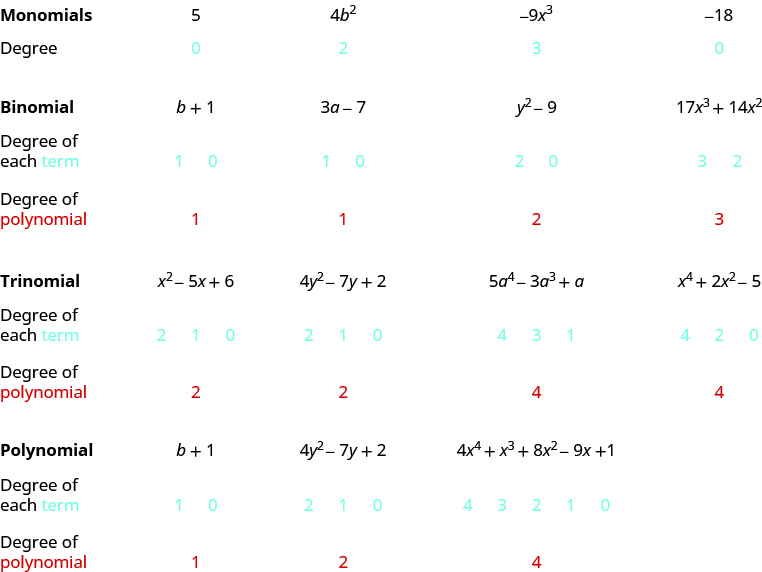## Identifying Characteristics of Polynomials

### Learning Outcomes

• Identify whether a polynomial is a monomial, binomial, or trinomial
• Determine the degree of a polynomial

## Identify Polynomials, Monomials, Binomials, and Trinomials

In Evaluate, Simplify, and Translate Expressions, you learned that a term is a constant or the product of a constant and one or more variables. When it is of the form $a{x}^{m}$, where $a$ is a constant and $m$ is a whole number, it is called a monomial. A monomial, or a sum and/or difference of monomials, is called a polynomial.

### Polynomials

polynomial—A monomial, or two or more monomials, combined by addition or subtraction
monomial—A polynomial with exactly one term
binomial— A polynomial with exactly two terms
trinomial—A polynomial with exactly three terms

Notice the roots:

• poly– means many
• mono– means one
• bi– means two
• tri– means three

Here are some examples of polynomials:

 Polynomial $b+1$ $4{y}^{2}-7y+2$ $5{x}^{5}-4{x}^{4}+{x}^{3}+8{x}^{2}-9x+1$ Monomial $5$ $4{b}^{2}$ $-9{x}^{3}$ Binomial $3a - 7$ ${y}^{2}-9$ $17{x}^{3}+14{x}^{2}$ Trinomial ${x}^{2}-5x+6$ $4{y}^{2}-7y+2$ $5{a}^{4}-3{a}^{3}+a$

Notice that every monomial, binomial, and trinomial is also a polynomial. They are special members of the family of polynomials and so they have special names. We use the words ‘monomial’, ‘binomial’, and ‘trinomial’ when referring to these special polynomials and just call all the rest ‘polynomials’.

### example

Determine whether each polynomial is a monomial, binomial, trinomial, or other polynomial:

1. $8{x}^{2}-7x - 9$
2. $-5{a}^{4}$
3. ${x}^{4}-7{x}^{3}-6{x}^{2}+5x+2$
4. $11 - 4{y}^{3}$
5. $n$

Solution

Polynomial Number of terms Type
1. $8{x}^{2}-7x - 9$ $3$ Trinomial
2. $-5{a}^{4}$ $1$ Monomial
3. ${x}^{4}-7{x}^{3}-6{x}^{2}+5x+2$ $5$ Polynomial
4. $11 - 4{y}^{3}$ $2$ Binomial
5. $n$ $1$ Monomial

### Determine the Degree of Polynomials

In this section, we will work with polynomials that have only one variable in each term. The degree of a polynomial and the degree of its terms are determined by the exponents of the variable.

A monomial that has no variable, just a constant, is a special case. The degree of a constant is $0$ —it has no variable.

### Degree of a Polynomial

The degree of a term is the exponent of its variable.
The degree of a constant is $0$.
The degree of a polynomial is the highest degree of all its terms.

Let’s see how this works by looking at several polynomials. We’ll take it step by step, starting with monomials, and then progressing to polynomials with more terms.

Remember: Any base written without an exponent has an implied exponent of $1$.### example

Find the degree of the following polynomials:

1. $4x$
2. $3{x}^{3}-5x+7$
3. $-11$
4. $-6{x}^{2}+9x - 3$
5. $8x+2$

Working with polynomials is easier when you list the terms in descending order of degrees. When a polynomial is written this way, it is said to be in standard form. Look back at the polynomials in the previous example. Notice that they are all written in standard form. Get in the habit of writing the term with the highest degree first.